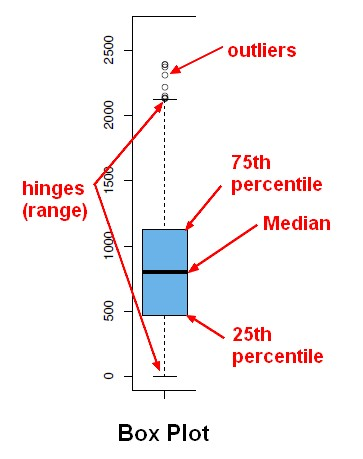# Quantile - Quartile

A quartile is a specialized quantile. It divides the data into 4 approximately equal groups.

## Percentile

The first and third quartile (or the 25th and 75th percentiles) are two very commonly used percentiles. Because they divide the data into quarters (when taken together with the median), they are referred to as the lower and upper quartiles. They are sometimes abbreviated Q_1 and Q_3.

Technically, the median is a “middle” quartile and is sometimes referred to as Q_2. Some also refer to the minimum value in a data set as Q_0 and the maximum as Q_4.

Quartile Abbreviations Description Percentile
Q_0 Minimum Value 0th percentile
Q_1 Lower quartile 25th percentile
Q_2 Middle quartile (median) 50th percentile
Q_3 Upper quartile 75th percentile
Q_4 Maximum Value 100th percentile

Discover MoreData Visualization - Box Plot

A box plot is a good summary of a distribution and was invented by John Tukey. See Five-number summary The boxplot is a special case of the quantile function in that it only returns the 1st, 2nd and...Distribution - Quantile Analysis

A quantile is a statistic that identifies the data that is less than the given value (ie that fall at or below a score in a distribution). A quantile function will always rank the data before giving any...Quantile - Ntile (N Quantile)

Ntile (ie Nquantile) The Ntile function bins a data set in bucket of equal frequency. Each bucket has the same amount of observations. 4tile is a quartile 100tile is a percentile